# Texas Go Math Grade 3 Lesson 17.3 Answer Key Find Unknown Side Lengths

Refer to our Texas Go Math Grade 3 Answer Key Pdf to score good marks in the exams. Test yourself by practicing the problems from Texas Go Math Grade 3 Lesson 17.3 Answer Key Find Unknown Side Lengths.

## Texas Go Math Grade 3 Lesson 17.3 Answer Key Find Unknown Side Lengths

Unlock the Problem

Chen has 27 feet of fencing to put around his garden. He has already used the lengths of fencing shown. How much fencing does he have left for the last side?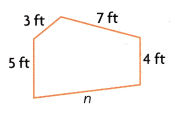Find the unknown side length.

Write an equation for the perimeter.
Think: If I knew the length of n, I would add all the side lengths to find the perimeter.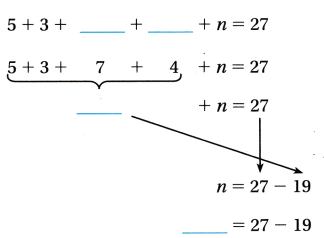Add the lengths of the sides you know.

Think: Addition and subtraction are inverse operations.

Write a related equation.
So, Chen has __________ feet of fencing left.
27-19=9 feet
Therefore, chen has 9 feet of fencing left.

Math Idea
A symbol or letter can stand for an unknown side length.

Example

Find unknown side lengths of a square.

The square has a perimeter of 20 inches. What is the length of each side of the square?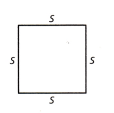Think: A square has four sides that are equal in length.
You can multiply to find the perimeter.

• Write a multiplication equation for the perimeter. 4 × s = 20
• Use a multiplication fact you know to solve. 4 × ________ = 20

So, the length of each side of the square is _______ inches.
5 inches

Explanation:
Multiplication equation for the perimeter 4 x s = 20
Multiplication fact to solve is 4 x 5 = 20
So, the length of each side of the square is 5 inches.

Example

Find unknown side lengths of a rectangle.

Lauren has a rectangular blanket. The perimeter is 28 feet. The width of the blanket is 5 feet. What is the length of the blanket?Add the lengths of the sides you know.
________ + ________ = _________
Subtract the lengths from the perimeter.
28 – ________ = ________
Divide the difference by 2.
_______ ÷ 2 = _________
So, the length of the blanket is ________ feet.
9 feet

Explanation:
Lauren has a rectangular blanket. The perimeter is 28 feet. The width of the blanket is 5 feet
The lengths of the sides i know is 5+5=10
Subtract the lengths from the perimeter, 28-10=18
Divide the difference by 2
18 ÷  2 = 9
So, the length of the blanket is 9 feet.

Share and Show

Find the unknown side lengths.

Question 1.
Perimeter = 25 centimetersn = _________ centimeters

Question 2.
Perimeter = 34 metersj = _________ meters
j = 10 meters

Explanation:
We know that perimeter of the rectangle is 34 meters
The sum of lengths of the sides i know is 7+7=14
Subtract the lengths from the perimeter, 34-14=20
Divide the difference by 2
20 ÷  2 = 10
The length of the other side is 10 meters
Therefore, j=10 meters

Question 3.
Perimeter = 12 feetr = __________ feet
r = 3 feet

Explanation:
We know that the perimeter of the given square is 12 feet
Think: A square has four sides that are equal in length.
You can multiply to find the perimeter.
Multiplication equation for the perimeter 4 x r = 12
Multiplication fact to solve is 4 x 3 = 12
So, the length of each side of the square is 3 feet

Math Talk
Mathematical Processes

Explain how you can use division to find the length a side of a square.
we have to divide the perimeter of a square by 4 to find the side of the square.

Unlock the Problem

Question 4.
Multi-Step Use Diagrams Latesha wants to make a border with ribbon around a figure she made and sketched at the right. She will use 44 centimeters of ribbon for the border. What is the unknown side length?(A) 3 centimeters
(B) 13 centimeters
(C) 9 centimeters
(D) 6 centimeters

a. What do you need to find?
I need to find the length of the missing side h.

I will subtract the sum of lengths of the sides given figure from the perimeter of the given figure.

c. Write an equation to solve the problem.
44 cm – 35cm = 9cm

d. Complete the sentences.
The perimeter is ________ centimeters.
The sum of the sides I know is ________ centimeters.
A related subtraction equation is
________ = ________ – ________
So, the length of side h is ________ centimeters.
The perimeter is 44 centimeters.
The sum of the sides I know is 35 centimeters.
A related subtraction equation is
h = 44 – 35
So, the length of side h is 9 centimeters.

e. Fill in the bubble for the correct answer choice above.
Option C. 9cm

Question 5.
H.O.T. Multi-Step A rectangle has a perimeter of 34 inches. The left side is 6 inches long. What is the length of the top side?11 inches

Explanation:
A rectangle has a perimeter of 34 inches. The left side is 6 inches long
The sum of lengths of the sides i know is 6+6=12
Subtract the lengths from the perimeter, 34-12=22
Divide the difference by 2
22 ÷  2 = 11
The length of the other side is 11 inches.

Question 6.
A wildlife sanctuary puts a fence around a section of their land. The fence is 19 kilometers long. What is the length of the unknown side?(A) 5 kilometers
(B) 7 kilometers
(C) 33 kilometers
(D) 14 kilometers
A. 5 kilometers.

Explanation:
The perimeter is 19 kilometers
The sum of the sides I know is 14 kilometers
A related subtraction equation is
n = 19-14
So, the length of side n is 5 kilometers

Question 7.
Ciara buys a piece of ribbon to sew around the edges of a square blanket. She uses 36 inches of ribbon. How long is each side of the blanket?
(A) 18 inches
(B) 9 inches
(C) 4 inches
(D) 32 inches
B. 9 inches

Explanation:
Ciara buys a piece of ribbon to sew around the edges of a square blanket. She uses 36 inches of ribbon
So, We came to know that the perimeter of the given square is 36 inches
Think: A square has four sides that are equal in length.
You can multiply to find the perimeter.
Multiplication equation for the perimeter 4 x r = 36
Multiplication fact to solve is 4 x 9 = 36
So, the length of each side of the square is 9 inches.
Therefore, each side of the blanket is 9 inches long.

Question 8.
Multi-Step Kenny puts a fence around his 5-sided garden. He starts with 35 feet of fencing. After he is done, he has 5 feet of fencing left over. How long is each side of his garden?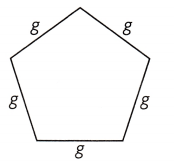(A) 7 feet
(B) 5 feet
(C) 6 feet
(D) 3 feet
C. 6 feet

Explanation:
Kenny puts a fence around his 5-sided garden. He starts with 35 feet of fencing. After he is done, he has 5 feet of fencing left over
35-5 = 30 feet
As given all the sides of the shape are same and perimeter is 30 feet
Multiplication equation for the perimeter 5 x g = 30 feet
Multiplication fact to solve is 5 x 6 = 30 feet
Therefore, the length od the side of the shape is 6 feet

Texas Test Prep

Question 9.
Eleni wants to put a fence around her square garden. The garden has a perimeter of 28 meters. How long will each side of the fence be?(A) 8 meters
(B) 7 meters
(C) 14 meters
(D) 6 meters
B. 7 feet

Explanation:
Eleni wants to put a fence around her square garden. The garden has a perimeter of 28 meters
We know that the perimeter of the given square is 28 meters
Think: A square has four sides that are equal in length.
You can multiply to find the perimeter.
Multiplication equation for the perimeter 4 x a = 28
Multiplication fact to solve is 4 x 7 = 28
So, the length of each side of the square is 7 meters

### Texas Go Math Grade 3 Lesson 17.3 Homework and Practice Answer Key

Find the unknown side lengths.

Question 1.
Perimeter = 28 centimetersm = __________ centimeters
m = 18 cm

Explanation:
We know that perimeter of the rectangle is 28 centimeters
The sum of lengths of the sides i know is 6+6=12
Subtract the lengths from the perimeter, 28-12=16
Divide the difference by 2
16 ÷  2 = 8
The length of the other side is 8 centimeters
Therefore, m=10 meters

Question 2.
Perimeter = 40 feet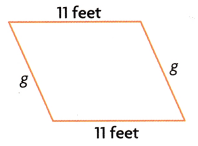g = __________ feet
g = 9 feet

Explanation:
We know that perimeter of the rectangle is 40 feet
The sum of lengths of the sides i know is 11+11=22
Subtract the lengths from the perimeter, 40-22=18
Divide the difference by 2
18 ÷  2 = 9
The length of the other side is 9 feet
Therefore, g=9 feet

Problem Solving

Use the diagram for 3-4.Question 3.
Mitch needs to replace the fence in his backyard. The diagram shows the lengths of the borders of the fence. The length of the fence is 62 meters. What is the unknown side length? Explain how you will solve the problem. Then write an equation to solve the problem.
x = 19 m

Explanation:
Mitch needs to replace the fence in his backyard. The diagram shows the lengths of the borders of the fence.
The length of the fence is 62 meters(perimeter)
The sum of the sides I know is 43 meters
A related subtraction equation is
x = 62-43 = 19
So, the length of side x is 19 meters

Question 4.
What if Mitch changes the shape of his fence to a rectangle? How much fencing will Mitch need? Explain.
52 meters

Explanation:
If Mitch changes the shape of his fence to a rectangle
Lengths od sides of rectangle are 14 and 12 meters
Perimeter of rectangle is 14+12+14+12=52
So, Mitch will need 52 meters of fencing.

Lesson Check

Question 5.
Nagi has a mirror in the shape of a hexagon. He puts a frame around it that has a perimeter of 42 inches.How long is each side?
(A) 7 inches
(B) 6 inches
(C) 8 inches
(D) 9 inches
A. 7 inches

Explanation:
Nagi has a mirror in the shape of a hexagon.
Nagi puts a frame around it that has a perimeter of 42 inches
Think: A hexagon has six sides that are equal in length.
You can multiply to find the perimeter.
Multiplication equation for the perimeter 6 x r = 42
Multiplication fact to solve is 6 x 7 = 42
So, the length of each side of the hexagon is 7 inches.

Question 6.
Zena has a square picture frame with a perimeter of 28 inches. Which equation can she use to find the length of each side?
(A) 4 × s = 28
(B) 4 + s = 28
(C) 28 × 4 = s
(D) 28 + 4 = s
A. 4 x s  = 28.

Explanation:
As we know that a square has 4 sides so, the perimeter of the square will be 4 x side.

Question 7.
A farmer is putting a new fence around a section of his ranch. The fence is 35 kilometers long. What is the length of the unknown side?(A) 6 kilometers
(B) 29 kilometers
(C) 7 kilometers
(D) 35 kilometers
A. 6 kilometers

Explanation:
A farmer is putting a new fence around a section of his ranch. The fence is 35 kilometers long
So, the perimeter is 35 kilometers
The sum of the sides I know is 29 kilometers
A related subtraction equation is
x = 35-29
So, the length of unknown side x is 6 kilometers.

Question 8.
Multi-Step Glenda puts a border of wallpaper around her bedroom wall. The room is a rectangle with side lengths of 3 meters and 4 meters. If she has 20 meters of wallpaper, how much wallpaper is left over?
(A) 13 meters
(B) 6 meters
(C) 27 meters
(D) 14 meters
B. 6 meters

Explanation:
Glenda puts a border of wallpaper around her bedroom wall.The room is a rectangle with side lengths of 3 meters and 4 meters
Perimeter of the room is 4+3+4+3=14 meters
If Glenda has 20 meters of wallpaper subtract 14 from 20
20-14 = 6 meters
Therefore, 6 meters of wallpaper is left with Glenda after bodering her bedroom.

Question 9.
Multi-Step Jorge sews a trim around the edge of a tablecloth with 8 sides. He has 32 feet of trim. If each side of the tablecloth is 2 feet long, how much trim will Jorge have left?
(A) 10 feet
(B) 8 feet
(C) 42 feet
(D) 16 feet